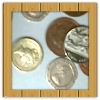#### You may also like### Coin Tossing Games

You and I play a game involving successive throws of a fair coin. Suppose I pick HH and you pick TH. The coin is thrown repeatedly until we see either two heads in a row (I win) or a tail followed by a head (you win). What is the probability that you win?### Win or Lose?

A gambler bets half the money in his pocket on the toss of a coin, winning an equal amount for a head and losing his money if the result is a tail. After 2n plays he has won exactly n times. Has he more money than he started with?A counter is placed in the bottom right hand corner of a grid. You toss a coin and move the star according to the following rules: ... What is the probability that you end up in the top left-hand corner of the grid?

# Mathsland National Lottery

### Why do this problem?

This problem offers an engaging context in which to develop students' understanding of experimental and theoretical probability.  The interactivity offers students a chance to get a 'feel' for the situation. Then they can calculate theoretical probabilities, perhaps by listing at first but then moving towards multiplying fractions based on conditional probabilities.

### Possible approach

Ideally, it would be good to have access to the Lottery Simulator interactivity as you introduce and work on this problem.  If this is not possible, you could simulate the lottery yourself by having numbered balls or digit cards in a bag.

To begin with, you could set up the interactivity so that six balls are available in the 'Number Tumbler' and 'your ticket' has three numbers.  Explain the way this lottery works to the group and invite suggestions for the numbers to choose on your ticket. Ask students to predict their chances of winning, and then simulate some draws - the simulator can be set to draw 10, 20, 50, 100 or even more draws.

"Did we win as often as you expected? How could we calculate the probability of winning?"
Give students some time to work on this. Some students may list combinations (systematically or otherwise), whereas others may use tree diagrams.

Bring the class together to share methods. Highlight anyone who has listed systematically to discuss the importance of making sure every combination is considered. If appropriate, move students towards a tree diagram approach, perhaps referring to the ideas in this article.

Move students on to the follow-up questions to consider the chances of winning with two or four balls (from six). Ideally, they will work on this using both listing and tree diagram approaches. Take time to discuss the symmetry that emerges from choosing a number on either side of three, and ask students to consider why this happens.
Working on this should give them enough confidence using tree diagrams to be able to answer the remaining follow-up questions without needing to list combinations.

### Key questions

Did we win as often as you expected?
How could we calculate the probability of winning?
Why is the probability of winning the two from six lottery the same as the probability of winning the four from six lottery?

### Possible extension

Students can be directed here to read about the UK National Lottery and see some animations based on real lottery statistics.

Students could consider the probability of matching two out of the three numbers they have chosen, and use the interactivity to compare experimental with theoretical probabilities. This extends to considering the UK National Lottery where prizes are awarded for matching 3, 4 or 5 out of the six numbers drawn.

Some students may wish to extend the 10-ball lottery question to the general case: what is the hardest lottery to win with $n$ balls? This could be a good opportunity to introduce them to factorial notation and the binomial coefficients.